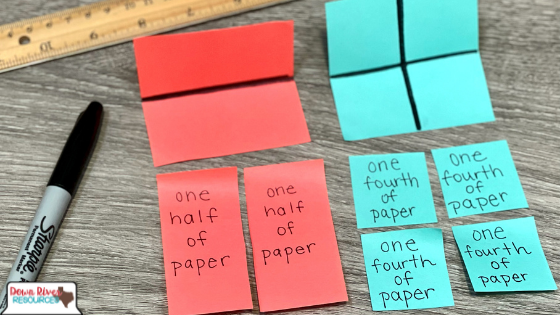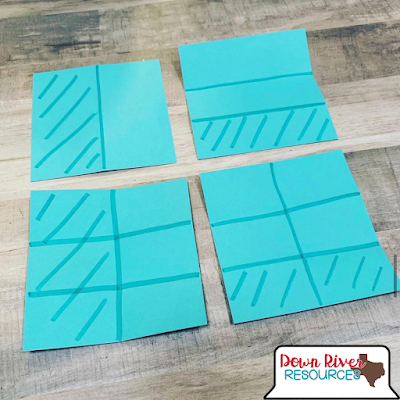Top 4 Ways to Use Paper Folding to Teach Fractions Conceptually - Down River Resources | Your Elementary Math Guide

# Top 4 Ways to Use Paper Folding to Teach Fractions Conceptually

Paper folding provides elementary mathematicians with the opportunity to visualize fractional size, and once stacked, allows students to see equivalency as a way of naming the same size area using a different number of units. There are several ways to use paper folding to visualize concepts that lead to fractional understanding including: comparing halves and fourths, generating equivalent fractions, adding fractions with unlike denominators, and subtracting fractions with unlike denominators. Here are my favorite ways to use paper folding to teach fractions conceptually:# The Best Ways to Teach Fractions for Understanding Using Paper Folding

## 1. Comparing Halves and Fourths

This paper folding activity is best used with mathematicians in first or second grades OR mathematicians who are struggling in upper grades.

➡️ Focus: Halves and Fourths

1️⃣ Fold one rectangular paper (copy paper or colored cardstock) in half. Then, cut the paper in half along the folded line.

2️⃣ Fold another rectangular paper in half and in half again. Then, cut the paper in fourths along the folded lines.

3️⃣ To support vocabulary development, write “one fourth of paper” on each of the four equal pieces of paper and “one half of paper” on each of the two equal pieces of paper.

4️⃣ After preparing the halves and the fourths, have one mathematician hold one of the halves and another mathematician hold one of the fourths.

5️⃣ Compare the pieces by holding them next to each other on on top of one another.

6️⃣ Ask: Who has the larger number of pieces? Who piece is largest?

These can be posted in the room as a reference, helping mathematicians visualize the concept of halves and fourths as well as the relationship between the two fractional parts.

#### This fraction paper folding activity focuses on following standards:

→ TEKS- 1.6G Partition two-dimensional figures into two and four fair shares or equal parts and describe the parts using words.

→ TEKS- 1.6H Identify examples and non-examples of halves and fourths.

→ TEKS 2.3A Partition objects into equal parts and name the parts, including halves, fourths, and eighths, using words.

## 2. Generating Equivalent Fractions with Paper Folding and Number Lines

This paper folding activity is best used with mathematicians in third grade OR mathematicians who are struggling in upper grades.

➡️ Focus: 1/2, 2/4, 3/6, and 4/8

1️⃣ Take a paper strip. Hold it horizontally. Fold it vertically down the middle.

Ask: How many equal parts are in the whole? Two. What fraction of the whole is one part? One half.

2️⃣ Draw a line to show where the paper was folded and label each half 1/2, one out of 2 units.

3️⃣ Repeat procedure and fold paper strips to show thirds, fourths, and fifths.

4️⃣ Draw a number line that is a little longer than the paper strip. Use your strip as a ruler to mark zero and 1 above the line, and 0/2 and 2/2 below the line.

5️⃣ Use the number line to generate equivalent fractions using the other paper strips.

#### This fraction paper folding activity focuses on following standards:

→ TEKS 3.3F Represent equivalent fractions with denominators of 2, 3, 4, 6, and 8 using a variety of objects and pictorial models, including number lines.

→ TEKS 3.3G Explain that two fractions are equivalent if and only if they are both represented by the same point on the number line or represent the same portion of a same size whole for an area model.

→ TEKS 4.3C Determine if two given fractions are equivalent using a variety of methods.

## 3. Adding Fractions with Unlike Denominators

This paper folding activity is best used with mathematicians in fourth and fifth grad mathematicians OR mathematicians who are struggling in middle school.

We lose a lot of mathematicians when introducing addends with unlike denominators.

➡️ Focus: 1/2 + 1/3

3️⃣ Share out with the class. Sample answer: “I cannot add one-half plus one-third until the units are the same. We need to find like units.”

Continue below for the rest of this activity.4️⃣ Make like units by folding paper as shown in the photograph above.

#### This fraction paper folding activity focuses on following standards:

→ TEKS 4.3D Compare two fractions with different numerators and different denominators and represent the comparison using the symbols >, =, or <.

→ TEKS 4.3E Represent and solve addition and subtraction of fractions with equal denominators using objects and pictorial models that build to the number line and properties of operations.

→ TEKS 5.3H Represent and solve addition and subtraction of fractions with unequal denominators referring to the same whole using objects and pictorial models and properties of operations.

## 4. Subtracting Fractions with Unlike Denominators

This paper folding activity is best used with mathematicians in fourth and fifth grad mathematicians OR mathematicians who are struggling in middle school.

Just like adding fractions with unlike denominators is tricky for mathematicians, so is subtraction!

➡️ Focus: 1/2 - 1/3

1️⃣ Ask: Can I subtract one-third from one-half?

3️⃣ Share out with the class. Sample answer: “I cannot subtract until the units are the same. We need to find like units.”

4️⃣ Draw one fraction model and partition it into two equal units. Then, write one-half below one part and shade. (This makes it easier to see one-half after changing the units.

5️⃣ Draw a second fraction model and partition it into thirds with horizontal lines. Then, write one-third below one part and shade.

6️⃣ Now make equivalent units.

7️⃣ Ask: How many new units do we have? Six units.

8️⃣ Ask: One half is how many sixths? One-half is three-sixths. One-third is how many sixths? One-third is two-sixths.

9️⃣ Write the equation with the number missing. Cross out 2 of the 3 shaded sixths. 3/6 - 2/6 = 1/6.  So, one-half minus one-third is one-sixth!

#### This fraction paper folding activity focuses on following standards:

→ TEKS 4.3D Compare two fractions with different numerators and different denominators and represent the comparison using the symbols >, =, or <.

→ TEKS 4.3E Represent and solve addition and subtraction of fractions with equal denominators using objects and pictorial models that build to the number line and properties of operations.

→ TEKS 5.3H Represent and solve addition and subtraction of fractions with unequal denominators referring to the same whole using objects and pictorial models and properties of operations.